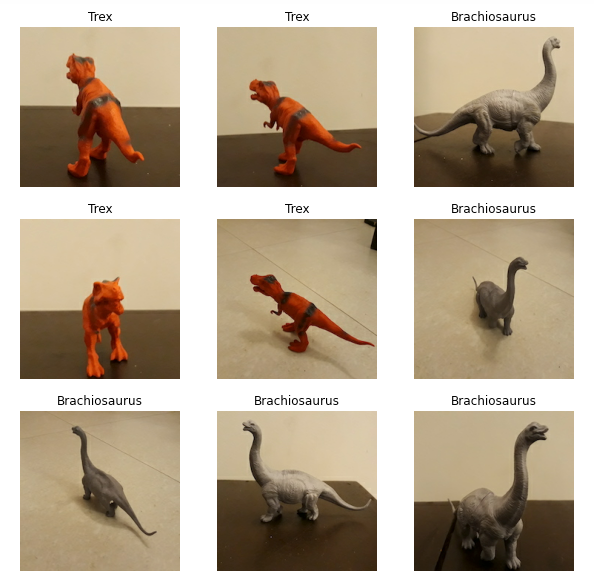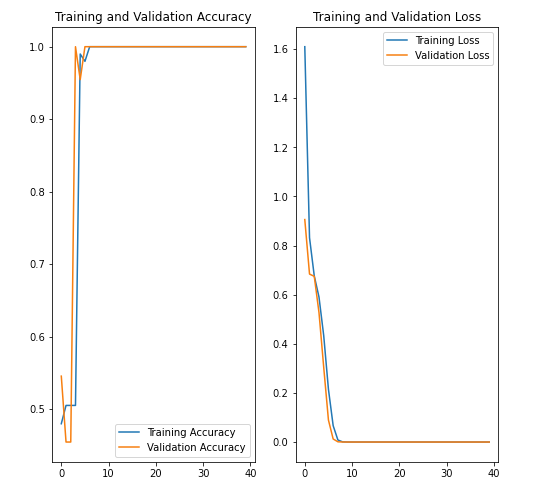# Building an image classifier with TensorFlow

I wanted to try solving a simple image classification problem using TensorFlow. My intention was to start everything from scratch, and cover all steps in the process of building a machine learning model including data preparation, model creation, training and finally using it for inference.

I was looking for two distinct objects for the project, and stumbled upon my son’s toy dinosaur collection. The red colour Tyrannosaurus (Trex) and the silver colour Brachiosaurus got my attention as good choices for the project.

## Data preparation

As with any other machine learning project, preparing the training data was the first step. The problem was much simpler in this case, as I was just going to train the model to classify between these two toys only. So, I thought that around 100 images of each object is enough to train the model.

To make things simpler I set my camera to capture photos with an aspect ratio of 1:1, or in other words, square images — with 2160 x 2160 pixels. When taking photos I made sure the dinos were captured from different angles. Moreover, I took them with two different backgrounds to remove unwanted bias towards the background in the trained model. Finally, I ended up taking 110 photos of each toy adding up to a total of 220 photos.

I organised the photos into two sub folders named after the respective dinosaur species. This was important as I was planning to use the image_dataset_from_directory method in Keras to create the dataset from the directory structure in disk. This method automatically labels the images inside each sub folder with the respective folder names.

Since this was a simpler problem to solve, I thought using high resolution images is an overkill. Therefore, I decided to resize all photos to be just 10% of their original size, and ended up with a bunch of 216 x 216 pixel images.

## Development environment

I already had a virtual environment configured with Python 3.8 and TensorFlow 2.4. With that I used a Jupyter notebook as it is much easier to code a project like this in a notebook.

I organised the sub folders containing the training images inside a parent folder called “Images”, and created a separate Test images folder for the photos I used for inference. Moreover, I created two notebook files — one for the data loading, model creation and training, whereas the other one for the inference.

## Importing the dependencies

Then it was time to do some coding!

Firstly I imported all the dependencies needed for the script.

`import matplotlib.pyplot as pltimport numpy as npimport osimport PILimport pathlibimport tensorflow as tffrom tensorflow import kerasfrom tensorflow.keras import layersfrom tensorflow.keras.models import Sequential`

## Creating the Datasets

I declared some global variables which were useful in my code. The path method in the pathlib helped me to access the files inside the Images folder via the data_dir variable. Since there were 220 images, I initially defined the batch size to be 55, which is just one fourth of the dataset. In addition, I declared two constants to hold the image height and width parameters. You will see these parameters in action later in this post.

`data_dir = pathlib.Path('Images')batch_size = 55image_height = 216image_width = 216`

I used the image_dataset_from_directory method in the Keras API to create and load the datasets. First I created the training dataset using 90% of the images with the help of the validation_split parameter. Since this method shuffles the dataset by default, I didn’t have to set the shuffle parameter explicitly.

Once executed successfully in Jupyter notebook, the method returned the following output.

Similarly, I created the validation dataset using the remaining 10% of the images as shown below.

## Exploring the datasets

I wanted to check whether the datasets have accurately derived the class names from the respective folder names and whether the images were loaded properly. I did this by executing the following code.

Here I read the training dataset and looped through the first 9 labels and images. The output looked like below.First 9 images and the respective labels in the training dataset

## Defining the model

Then it was time to create the model!

I used the Sequential class of Keras API to group the layers sequentially in this model. A sequential model is more appropriate for a simple problem like this as I was not going to deal with multiple inputs or outputs in the model, or its layers.

I defined the model with a very basic architecture having just 3 convolution layers each followed by a max pooling layer. The output from the last convolution and max pooling layers was flattened and fed into a fully connected layer with 128 neurons. This layer was followed by the final layer having just 2 nodes representing the two object classes to predict.

The code for the model is as follows.

You may notice here that I have rescaled the images initially using a separate layer before feeding into the first convolution layer. The pixel data is typically in the RGB channel value range which is 0–255. The idea behind this rescaling was to standardise the pixel data by transforming each value into its corresponding value in the range 0–1.

The summary of the layers could be viewed using the following code.

`model.summary()`

## Compiling the model

After defining the model it was then time to compile it.

Here I used the Adam optimisation method and the Sparse Categorical Crossentropy loss function for the model.

## Training the model

The fit method was used to train the model and I passed the training dataset, the validation dataset and the number of epochs into it. After that, I ran the training for 40 epochs.

The model started achieving 100% training and validation accuracy just after executing less than 10 epochs. This immediately suggested that I could have trained this model without sparing 40 epochs.

## Visualising training performance

I used the following code to plot two graphs and visualise the overall training performance. The first one showed the training and validation accuracy whereas the other one showed the training and validation losses against the number of epochs executed.Training and validation accuracy and the corresponding losses against the epochs executed

## Using the model for inference

Then came the fun part of using the model for inference!

Since the model in this case was meant only for classifying images of the two specific toys, I used some photos of them which weren’t used in the training or the validation sets before.

I wrote the following code to print the test images together with the corresponding predictions by the model. Each prediction was displayed as a combination of the name of the dinosaur and the percentage confidence.